Subsections

# Model

## Hamiltonian and Partition Function

We have N spins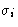, i=1, 2,... on a lattice with coordinate number z. Each spin can be either up or down: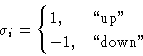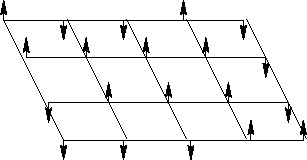#### Energy:

1.
Each spin interacts with external field H: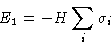2.
Each pair of neighbors interacts:
(a)
Parallel spins are favored--energy -J, J>0
(b)
Antiparallel spins are discouraged--energy +J
This can be written as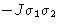. The total interaction: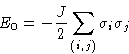Here (i,j) means sum over all pairs of nearest neighbors
Result: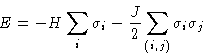(1)

## Partition Function and Thermodynamic Variables

Microscopic state of the system--combination of all spins: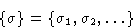Partition function: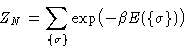We don't have momenta here--this is actually a configuration integral rather than partition function! Free energy: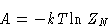Average magnetization (H is conjugated to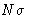!)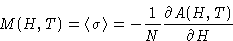Susceptibility: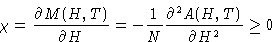Energy: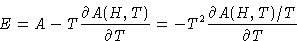Specific heat: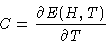© 1997 Boris Veytsman and Michael Kotelyanskii
Mon Oct 13 22:07:20 EDT 1997